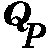#Quantum Probability

Quantum probability is a mathematical framework for calculating probabilities, just like Kolmogorov's well-known axiomatic scheme. But unlike the latter it allows for random variables which can not be measured together, such as position and momentum in Heisenberg's uncertainty principle. The building blocks of quantum probability are operators on a Hilbert space. Its goal is to extend the good ideas in probability theory to the quantum regime, such as independence, random walk, Brownian motion, Markov chain, ergodicity, recurrence and transience, martingales and the like.
In Nijmegen we specialise in applications to quantum optics and ergodic theory of quantum measurement.

Work in Quantum Probability is disseminated through the book series

## Quantum Probability Communications

World Scientific, eds. J.M. Lindsay and S. Attal.
The volumes XI and XII in particular are intended as introductory material in this field.